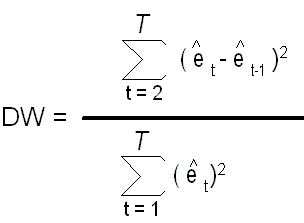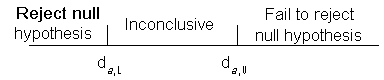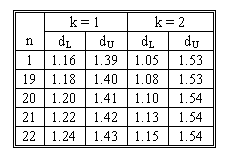### Why should I choose AnalystNotes?

AnalystNotes specializes in helping candidates pass. Period.

##### Subject 12. The Durbin-Watson Statistic
The most popular test for serial correlation is the Durbin-Watson test. This test is designed to identify the statistical significance of any positive serial correlation within the regression residuals.

The Durbin-Watson test statistics d is defined as follows:Where e-hatt is the regression residual for period t.

If the errors are homoskedastic and not serially correlated, then the Durbin-Watson statistic will be close to 2. Therefore, we can test the null hypothesis that the errors are not serially correlated by testing whether the Durbin-Watson statistic is significantly different from 2.

If the sample is very large, the Durbin-Watson statistic will be approximately equal to 2 (1 - r), where r is the sample correlation between the regression residuals from one period and those from the previous period. The approximation shows the value of the Durbin-Watson statistic for differing levels of serial correlation.

• If r = 1, then d = 0: this indicates positive correlation.
• If r = 0, then d = 2: this supports the null hypothesis of no serial correlation.
• If r = -1, then d = 4: this indicates negative correlation.

The test statistic d is a random variable whose value varies from sample to sample. The sampling distribution of d is quite complicated and depends on the values assumed by the explanatory variables. As a result, the sampling distribution of d changes from one problem to another, and no single distribution can be tabulated to provide critical values. However, Durbin and Watson found bounds that hold for all data sets, and these bounds have been tabulated.

To test for positive serial correlation, the critical region falls in the left tail of the d distribution. Let dα denote the critical value dα such that the probability of obtaining a d statistic less than dα is equal to the level of significance. That is:

P(d < dα) = α

Our decision rule is

Reject H0: r = 0 in favor of H1: r > 0 if d < dα

For a given value α, the critical value dα is unknown, but Durbin and Watson found that this critical value must lie between two values called the lower bound dα, L and upper bound dα, U. The lower and upper bounds depend on the sample size n, the number of explanatory variables included in the regression equation K, and the level of significance of the test α. Thus we have, for a given level of significance α,

dα, L ≤ dα ≤ dα, U

To determine the statistical significance of the serial correlation, we compute a value for the Durbin-Watson test statistic and compare this figure to the two known values (dα, L and dα, U)rather than to the unknown dα.

• If the Durbin-Watson statistic is greater than the upper boundary, then you should fail to reject the null hypothesis.
• If the Durbin-Watson statistic is less than the lower boundary, then the null hypothesis should be rejected.
• If the Durbin-Watson statistic lies between the upper and lower boundary, then the results of the test are inconclusive at the given level of significance. In other words, when the Durbin-Watson statistic lies between the two critical values, then we can neither support nor refute the claim that no statistically significant serial correlation exists within the regression residuals.Suppose we want to test H0: r = 1 against H1: r > 0 at the 5% level of significance using the Durbin-Watson test. Assume that there are 20 observations and K = 1 explanatory variable. Find the lower and upper bounds dα, L and dα, U.

From the table below the appropriate values are d0.05, L = 1.20 and d0.05, U = 1.41. Thus we should reject H0 if d < 1.20, not reject H0 if d > 1.41, and withhold judgment if 1.20 ≤ d ≤ 1.41.

Significance points of dL and dU: 5%Learning Outcome Statements

k. explain the types of heteroskedasticity and how heteroskedasticity and serial correlation affect statistical inference;

CFA® 2023 Level I Curriculum, Volume 1, Module 2